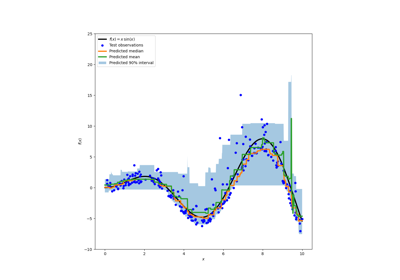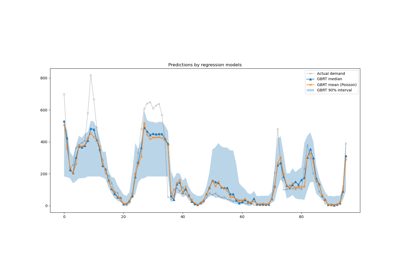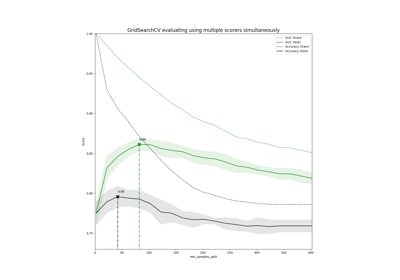# sklearn.metrics.make_scorer¶

sklearn.metrics.make_scorer(score_func, *, response_method=None, greater_is_better=True, needs_proba='deprecated', needs_threshold='deprecated', **kwargs)[source]

Make a scorer from a performance metric or loss function.

A scorer is a wrapper around an arbitrary metric or loss function that is called with the signature scorer(estimator, X, y_true, **kwargs).

It is accepted in all scikit-learn estimators or functions allowing a scoring parameter.

The parameter response_method allows to specify which method of the estimator should be used to feed the scoring/loss function.

Read more in the User Guide.

Parameters:
score_funccallable

Score function (or loss function) with signature score_func(y, y_pred, **kwargs).

response_method{“predict_proba”, “decision_function”, “predict”} or list/tuple of such str, default=None

Specifies the response method to use get prediction from an estimator (i.e. predict_proba, decision_function or predict). Possible choices are:

• if str, it corresponds to the name to the method to return;

• if a list or tuple of str, it provides the method names in order of preference. The method returned corresponds to the first method in the list and which is implemented by estimator.

• if None, it is equivalent to "predict".

New in version 1.4.

greater_is_betterbool, default=True

Whether score_func is a score function (default), meaning high is good, or a loss function, meaning low is good. In the latter case, the scorer object will sign-flip the outcome of the score_func.

needs_probabool, default=False

Whether score_func requires predict_proba to get probability estimates out of a classifier.

If True, for binary y_true, the score function is supposed to accept a 1D y_pred (i.e., probability of the positive class, shape (n_samples,)).

Deprecated since version 1.4: needs_proba is deprecated in version 1.4 and will be removed in 1.6. Use response_method="predict_proba" instead.

needs_thresholdbool, default=False

Whether score_func takes a continuous decision certainty. This only works for binary classification using estimators that have either a decision_function or predict_proba method.

If True, for binary y_true, the score function is supposed to accept a 1D y_pred (i.e., probability of the positive class or the decision function, shape (n_samples,)).

For example average_precision or the area under the roc curve can not be computed using discrete predictions alone.

Deprecated since version 1.4: needs_threshold is deprecated in version 1.4 and will be removed in 1.6. Use response_method=("decision_function", "predict_proba") instead to preserve the same behaviour.

Additional parameters to be passed to score_func.

Returns:
scorercallable

Callable object that returns a scalar score; greater is better.

Examples

>>> from sklearn.metrics import fbeta_score, make_scorer
>>> ftwo_scorer = make_scorer(fbeta_score, beta=2)
>>> ftwo_scorer
make_scorer(fbeta_score, response_method='predict', beta=2)
>>> from sklearn.model_selection import GridSearchCV
>>> from sklearn.svm import LinearSVC
>>> grid = GridSearchCV(LinearSVC(), param_grid={'C': [1, 10]},
...                     scoring=ftwo_scorer)


## Examples using sklearn.metrics.make_scorer¶Prediction Intervals for Gradient Boosting Regression

Prediction Intervals for Gradient Boosting RegressionLagged features for time series forecasting

Lagged features for time series forecastingDemonstration of multi-metric evaluation on cross_val_score and GridSearchCV

Demonstration of multi-metric evaluation on cross_val_score and GridSearchCV